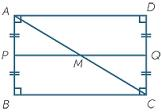# Rectangular 6255

The lengths of the sides of the rectangular garden are in the ratio of 1: 2. The connection of the centers of the adjacent sides is 20 m long. Calculate the perimeter and area of the rectangle.

o =  107.3313 m
S =  640 m2

### Step-by-step explanation:Did you find an error or inaccuracy? Feel free to write us. Thank you!

Tips for related online calculators
Are you looking for help with calculating roots of a quadratic equation?
Check out our ratio calculator.

#### You need to know the following knowledge to solve this word math problem:

We encourage you to watch this tutorial video on this math problem: